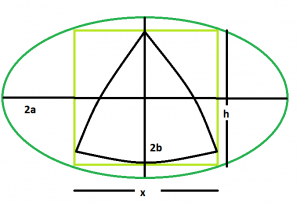# Biggest Reuleaux Triangle inscribed within a square which is inscribed within an ellipse

Given an ellipse with major axis length and minor axis 2a & 2b respectively which inscribes a square which in turn inscribes a reuleaux triangle. The task is to find the maximum possible area of this reuleaux triangle.

Examples:

```Input: a = 5, b = 4
Output: 0.0722389

Input: a = 7, b = 11
Output: 0.0202076
```

## Recommended: Please try your approach on {IDE} first, before moving on to the solution.Approach: As, the side of the square inscribed within an ellipse is, x = √(a^2 + b^2)/ab. Please refer Area of the Largest square that can be inscribed in an ellipse.
Also, in the reuleaux triangle, h = x = √(a^2 + b^2)/ab.
So, Area of the reuleaux triangle, A = 0.70477*h^2 = 0.70477*((a^2 + b^2)/a^2b^2).

Below is the implementation of the above approach:

## C++

 `// C++ Program to find the biggest Reuleaux triangle ` `// inscribed within in a square which in turn ` `// is inscribed within an ellipse ` `#include ` `using` `namespace` `std; ` ` `  `// Function to find the biggest reuleaux triangle ` `float` `Area(``float` `a, ``float` `b) ` `{ ` ` `  `    ``// length of the axes cannot be negative ` `    ``if` `(a < 0 && b < 0) ` `        ``return` `-1; ` ` `  `    ``// height of the reuleaux triangle ` `    ``float` `h = ``sqrt``(((``pow``(a, 2) + ``pow``(b, 2)) ` `                    ``/ (``pow``(a, 2) * ``pow``(b, 2)))); ` ` `  `    ``// area of the reuleaux triangle ` `    ``float` `A = 0.70477 * ``pow``(h, 2); ` ` `  `    ``return` `A; ` `} ` ` `  `// Driver code ` `int` `main() ` `{ ` `    ``float` `a = 5, b = 4; ` `    ``cout << Area(a, b) << endl; ` ` `  `    ``return` `0; ` `} `

## Java

 `// Java Program to find the biggest Reuleaux triangle ` `// inscribed within in a square which in turn ` `// is inscribed within an ellipse ` `import` `java.io.*; ` ` `  `class` `GFG  ` `{ ` `     `  `// Function to find the biggest reuleaux triangle ` `static` `float` `Area(``float` `a, ``float` `b) ` `{ ` ` `  `    ``// length of the axes cannot be negative ` `    ``if` `(a < ``0` `&& b < ``0``) ` `        ``return` `-``1``; ` ` `  `    ``// height of the reuleaux triangle ` `    ``float` `h = (``float``)Math.sqrt(((Math.pow(a, ``2``) + Math.pow(b, ``2``)) ` `                ``/ (Math.pow(a, ``2``) * Math.pow(b, ``2``)))); ` ` `  `    ``// area of the reuleaux triangle ` `    ``float` `A = (``float``)(``0.70477` `* Math.pow(h, ``2``)); ` ` `  `    ``return` `A; ` `} ` ` `  `// Driver code ` `public` `static` `void` `main (String[] args) ` `{ ` `    ``float` `a = ``5``, b = ``4``; ` `    ``System.out.println(Area(a, b)); ` `} ` `} ` ` `  `// This code is contributed by anuj_67.. `

## Python3

 `# Python3 Program to find the biggest Reuleaux  ` `# triangle inscribed within in a square ` `# which in turn is inscribed within an ellipse  ` `import` `math; ` ` `  `# Function to find the biggest  ` `# reuleaux triangle  ` `def` `Area(a, b): ` ` `  `    ``# length of the axes cannot  ` `    ``# be negative  ` `    ``if` `(a < ``0` `and` `b < ``0``):  ` `        ``return` `-``1``;  ` ` `  `    ``# height of the reuleaux triangle  ` `    ``h ``=` `math.sqrt(((``pow``(a, ``2``) ``+` `pow``(b, ``2``)) ``/` `                   ``(``pow``(a, ``2``) ``*` `pow``(b, ``2``))));  ` ` `  `    ``# area of the reuleaux triangle  ` `    ``A ``=` `0.70477` `*` `pow``(h, ``2``);  ` ` `  `    ``return` `A;  ` ` `  `# Driver code  ` `a ``=` `5``; ` `b ``=` `4``;  ` `print``(``round``(Area(a, b), ``7``)); ` ` `  `# This code is contributed by chandan_jnu `

## C#

 `// C# Program to find the biggest Reuleaux triangle ` `// inscribed within in a square which in turn ` `// is inscribed within an ellipse ` `using` `System; ` ` `  `class` `GFG  ` `{ ` `     `  `// Function to find the biggest reuleaux triangle ` `static` `double` `Area(``double` `a, ``double` `b) ` `{ ` ` `  `    ``// length of the axes cannot be negative ` `    ``if` `(a < 0 && b < 0) ` `        ``return` `-1; ` ` `  `    ``// height of the reuleaux triangle ` `    ``double` `h = (``double``)Math.Sqrt(((Math.Pow(a, 2) +  ` `                                    ``Math.Pow(b, 2)) / ` `                                   ``(Math.Pow(a, 2) *  ` `                                   ``Math.Pow(b, 2)))); ` ` `  `    ``// area of the reuleaux triangle ` `    ``double` `A = (``double``)(0.70477 * Math.Pow(h, 2)); ` ` `  `    ``return` `A; ` `} ` ` `  `// Driver code ` `static` `void` `Main() ` `{ ` `    ``double` `a = 5, b = 4; ` `    ``Console.WriteLine(Math.Round(Area(a, b),7)); ` `} ` `} ` ` `  `// This code is contributed by chandan_jnu `

## PHP

 ` `

Output:

```0.0722389
```

Attention reader! Don’t stop learning now. Get hold of all the important DSA concepts with the DSA Self Paced Course at a student-friendly price and become industry ready.

My Personal Notes arrow_drop_upCheck out this Author's contributed articles.

If you like GeeksforGeeks and would like to contribute, you can also write an article using contribute.geeksforgeeks.org or mail your article to contribute@geeksforgeeks.org. See your article appearing on the GeeksforGeeks main page and help other Geeks.

Please Improve this article if you find anything incorrect by clicking on the "Improve Article" button below.

Improved By : vt_m, Chandan_Kumar, AnkitRai01